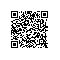# C++第11周项目3（4）——万以内回文数

【项目3- 有趣的数字】先阅读例题，体会处理数字的一般方法，然后自行选题进行解决，掌握这种类型程序设计的一般方法。

4）输出10000以内的所有回文数。

#include<iostream>
using namespace std;
int main()
{

int m,n,k,c=0;
cout<<"一万内的回文数："<<endl;
for(m=1;m<=10000;++m)
{
k=m;
n=0;
while(k>0)
{
n=n*10+k%10;
k=k/10;
}
if(m==n)
{
++c;
cout<<m<<"\t";
if(c%10==0)
cout<<endl;
}
}
cout<<endl;
return 0;
}#include<iostream>
using namespace std;
int main()
{

int m,n;
cout<<"一万内的回文数："<<endl;
//个位数全是回文数
for(m=1;m<=9;++m)
cout<<m<<"\t";
//下面凑出所有两位的回文数
for(m=1;m<=9;++m)
cout<<(m*10+m)<<"\t";
//下面凑是所有三位的回文数
for(m=1;m<=9;++m)
for(n=0;n<=9;++n)
cout<<(m*100+n*10+m)<<"\t";
//下面凑出所有四位的回文数
for(m=10;m<=99;++m)
{
n=(m%10)*10+m/10; //n和m比，个位、十位恰互换
cout<<(m*100+n)<<"\t";
}
cout<<endl;
return 0;
}使用钉钉扫一扫加入圈子
+ 订阅